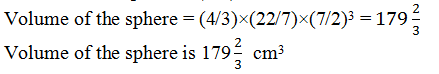Guru

# Find the volume of a sphere whose surface area is 154 cm2. (Assume π = 22/7) Q.7

• 0

sir what is the best way for solving the question of class 9th chapter of Surface areas and Volumes chapter of question no. of math of exercise 13.8   of math, what is the best way for solving the question of class 9th ncert book of question no.7

Share

1. Let r be the radius of a sphere.

Surface area of sphere = 4πr2

4πr2 = 154 cm2 (given)

r2 = (154×7)/(4 ×22)

r = 7/2